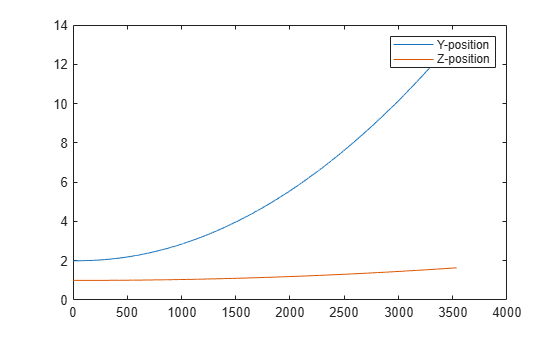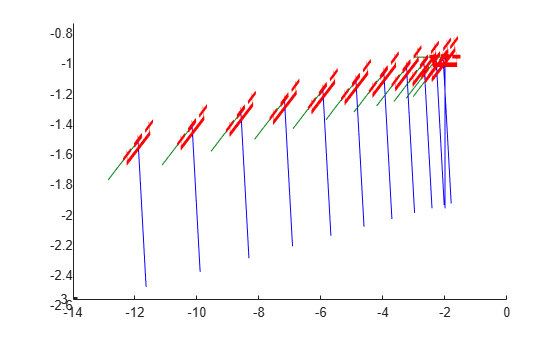Main Content

# multirotor

Guidance model for multirotor UAVs

## Description

A `multirotor` object represents a reduced-order guidance model for an unmanned aerial vehicle (UAV). The model approximates the behavior of a closed-loop system consisting of an autopilot controller and a multirotor kinematic model for 3-D motion.

For fixed-wing UAVs, see `fixedwing`.

## Creation

`model = multirotor` creates a multirotor motion model with `double` precision values for inputs, outputs, and configuration parameters of the guidance model.

`model = multirotor(DataType)` specifies the data type precision (`DataType` property) for the inputs, outputs, and configurations parameters of the guidance model.

## Properties

expand all

Name of the UAV, used to differentiate it from other models in the workspace, specified as a string scalar.

Example: `"myUAV1"`

Data Types: `string`

UAV controller configuration, specified as a structure of parameters. Specify these parameters to tune the internal control behaviour of the UAV. Specify the proportional (P) and derivative (D) gains for the dynamic model and other UAV parameters. For multirotor UAVs, the structure contains these fields with defaults listed:

• `'PDRoll'`- `[3402.97 116.67]`

• `'PDPitch'` - `[3402.97 116.67]`

• `'PYawRate'` - `1950`

• `'PThrust'` - `3900`

• `'Mass'` - `0.1` (measured in kg)

Example: ```struct('PDRoll',[3402.97,116.67],'PDPitch',[3402.97, 116.67],'PYawRate',1950,'PThrust',3900,'Mass',0.1)```

Data Types: `struct`

This property is read-only.

UAV guidance model type, specified as `'MultirotorGuidance'`.

Input and output numeric data types, specified as either `'double'` or `'single'`. Choose the data type based on possible software or hardware limitations. Specify `DataType` when first creating the object.

## Object Functions

 `control` Control commands for UAV `derivative` Time derivative of UAV states `environment` Environmental inputs for UAV `state` UAV state vector

## Examples

collapse all

This example shows how to use the `multirotor` guidance model to simulate the change in state of a UAV due to a command input.

Create the multirotor guidance model.

`model = multirotor;`

Create a state structure. Specify the location in world coordinates.

```s = state(model); s(1:3) = [3;2;1];```

Specify a control command, `u`, that specified the roll and thrust of the multirotor.

```u = control(model); u.Roll = pi/12; u.Thrust = 1;```

Create a default environment without wind.

`e = environment(model);`

Compute the time derivative of the state given the current state, control command, and environment.

`sdot = derivative(model,s,u,e);`

Simulate the UAV state using `ode45` integration. The `y` field outputs the multirotor UAV states as a 13-by-n matrix.

```simOut = ode45(@(~,x)derivative(model,x,u,e), [0 3], s); size(simOut.y)```
```ans = 1×2 13 3536 ```

Plot the change in roll angle based on the simulation output. The roll angle (the X Euler angle) is the 9th row of the `simOut.y` output.

`plot(simOut.y(9,:))`Plot the change in the Y and Z positions. With the specified thrust and roll angle, the multirotor should fly over and lose some altitude. A positive value for Z is expected as positive Z is down.

```figure plot(simOut.y(2,:)); hold on plot(simOut.y(3,:)); legend('Y-position','Z-position') hold off```You can also plot the multirotor trajectory using `plotTransforms`. Create the translation and rotation vectors from the simulated state. Downsample (every 300th element) and transpose the `simOut` elements, and convert the Euler angles to quaternions. Specify the mesh as the `multirotor.stl` file and the positive Z-direction as `"down"`. The displayed view shows the UAV translating in the Y-direction and losing altitude.

```translations = simOut.y(1:3,1:300:end)'; % xyz position rotations = eul2quat(simOut.y(7:9,1:300:end)'); % ZYX Euler plotTransforms(translations,rotations,... 'MeshFilePath','multirotor.stl','InertialZDirection',"down") view([90.00 -0.60])```## More About

expand all

 Mellinger, Daniel, and Nathan Michael. "Trajectory Generation and Control for Precise Aggressive Maneuvers with Quadrotors." The International Journal of Robotics Research. 2012, pp. 664-74.

## SupportGet trial now